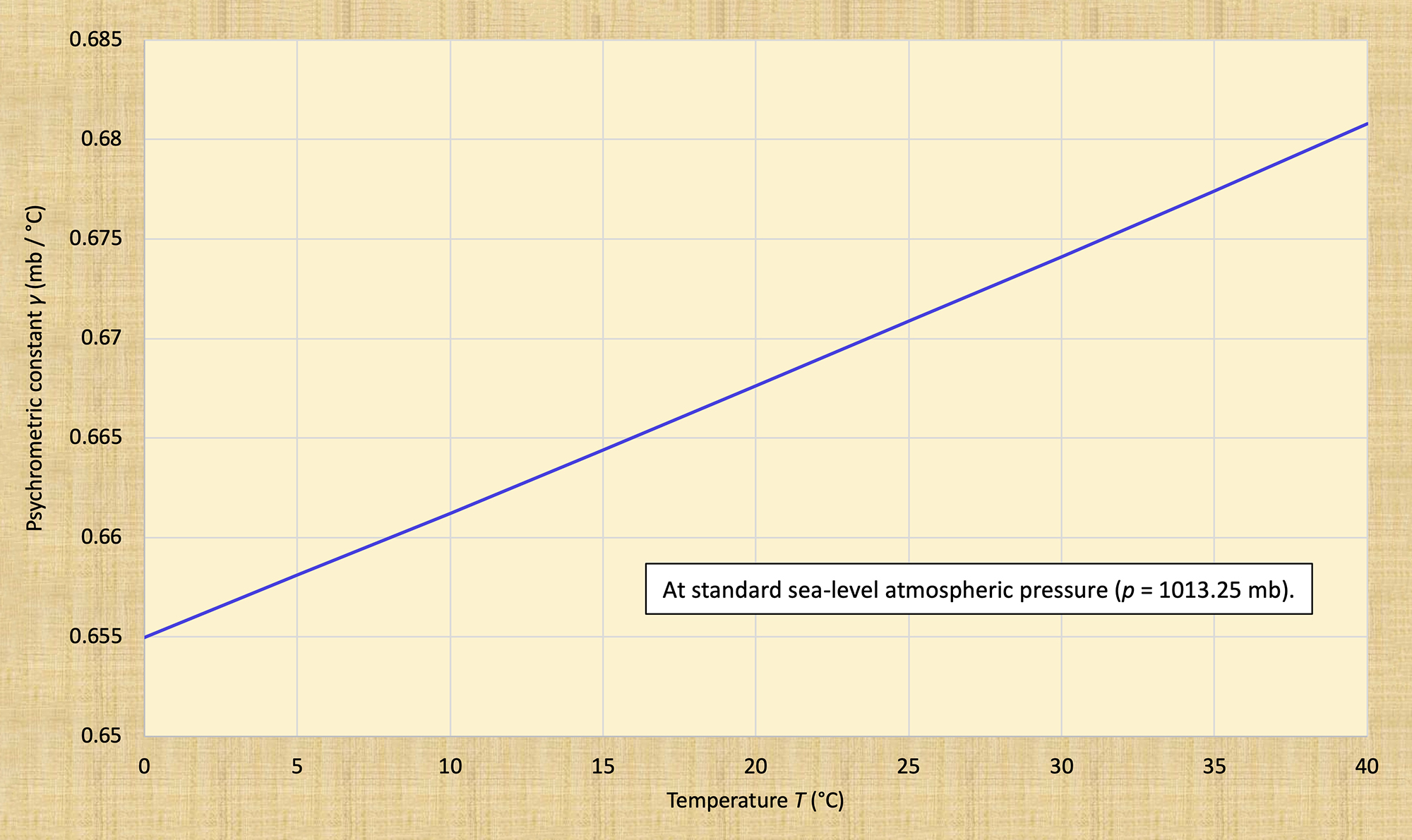The psychrometric constant Victor M. Ponce11 March 2014

 Abstract. The slight variation of the psychrometric constant γ with temperature has been explained and clarified. The aim is to increase the accuracy of online evaporation calculations.

1.  Bowen ratio

The Bowen ratio is defined as follows (Ponce, 1989):

 Qh                   Ts  -  Ta             p B  =   ______   =  γ   ____________   ________            Qe                   es  -  ea          1000 (1)

in which B = Bowen ratio; Qh = sensible heat transfer from water body to the atmosphere by convection and conduction; Qe = energy expended in the evaporation process; Ts = water surface temperature, in °C; Ta = overlying air temperature, in °C; es = saturation vapor pressure at the water surface temperature, in millibars (mb); ea = vapor pressure of the overlying air, in mb; p = atmospheric pressure, in mb; γ = psychrometric constant, in mb °C-1 (Note that 1000 has the units of mb).

2.  The psychrometric constant γ

The psychrometric constant γ is expressed as follows:

 cp p γ  =  __________              rMW λ (2)

in which cp = specific heat of air at constant pressure, in cal gr-1 °C-1; p = atmospheric pressure, in mb; rMW = ratio of the molecular weight of water vapor to dry air: rMW = 0.622, and λ = latent heat of water vaporization, in cal gr-1.

The specific heat of air between 0°C and 40°C is: cp = 1.005 J gr-1 °C-1 = 0.24017 cal gr-1 °C-1. The mean sea-level atmospheric pressure is: p = 1013.25 mb. Therefore, at sea level, the psychrometric constant is:

 (0.24017) (1013.25) γ  =  ___________________________                       0.622 λ (3)

which reduces to:

 391.24 γ  =  ___________                  λ (4)

with λ in cal gr-1 and γ in mb °C-1.

For any atmospheric pressure p (mb), the psychrometric constant is:

 0.24017 p γ  =  ________________                0.622 λ (5)

which reduces to:

 0.3861 p γ  =  _______________                     λ (6)

with p in mb, λ in cal gr-1 and γ in mb °C-1.

3.  Variation of γ with temperature

Since λ varies with temperature, γ also varies with temperature. Table 1 shows the variation of the psychrometric constant γ with temperature, at standard sea-level atmospheric pressure (p = 1013.25 mb) (Fig. 1).

 Table 1.  Variation of psychrometric constant γ with temperature,at standard atmospheric pressure. T(°C) λ(cal gr-1) γ(mb °C-1) 0 597.3 0.655 5 594.5 0.658 10 591.7 0.661 15 588.9 0.664 20 586.0 0.668 25 583.2 0.671 30 580.4 0.674 35 577.6 0.677 40 574.7 0.681Fig. 1  Variation of the psychrometric constant with temperature,
at standard atmospheric pressure.

4.  Summary

The slight variation of the psychrometric constant γ with temperature has been explained and clarified. The aim is to increase the accuracy of online evaporation calculations (http://onlinecalc.sdsu.edu)Fig. 2  Ira Sprague Bowen.

References

Ponce, V. M. 1989. Engineering Hydrology: Principles and Practices. Prentice Hall, Englewood Cliffs, New Jersey.

 190823